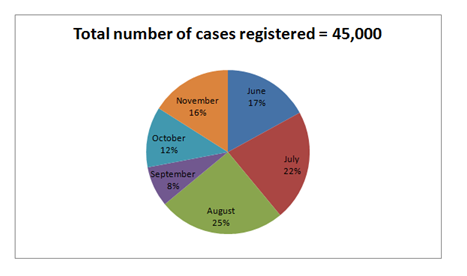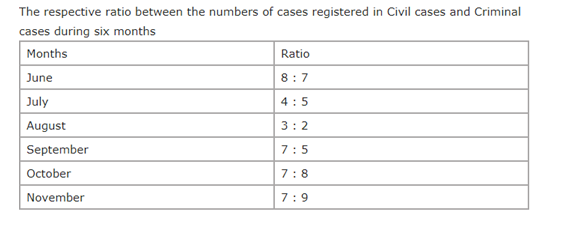# “20-20” Quantitative Aptitude | Crack SBI Clerk 2018 Day-25

Dear Friends, SBI Clerk 2018 Notification has been released we hope you all have started your preparation. Here we have started New Series of Practice Materials specially for SBI Clerk 2018. Aspirants those who are preparing for the exams can use this “20-20” Quantitative Aptitude Questions.

## Daily Practice Test Schedule | Good Luck

 Topic Daily Publishing Time Daily News Papers & Editorials 8.00 AM Current Affairs Quiz 9.00 AM Logical Reasoning 10.00 AM Quantitative Aptitude “20-20” 11.00 AM Vocabulary (Based on The Hindu) 12.00 PM Static GK Quiz 1.00 PM English Language “20-20” 2.00 PM Banking Awareness Quiz 3.00 PM Reasoning Puzzles & Seating 4.00 PM Daily Current Affairs Updates 5.00 PM Data Interpretation / Application Sums (Topic Wise) 6.00 PM Reasoning Ability “20-20” 7.00 PM English Language (New Pattern Questions) 8.00 PM General / Financial Awareness Quiz 9.00 PM

[WpProQuiz 1304]

Click “Start Quiz” to attend these Questions and view Explanation

### Click Here for SBI Clerk Prelims and Mains 2018 – Full Length Mock Test

1. A sum of money invested for 7 years in Scheme 1 which offers SI at a rate of 8% pa. The amount received from Scheme 1 after 7 years invested for 2 years in Scheme 2 which offers CI rate of 10% pa. If the interest received from Scheme B was Rs.1638. What was the sum invested in Scheme 1 ?
1. Rs.7500
2. Rs.5000
3. Rs.8200
4. Rs.9000
5. None of these
1. If the numerator of a fraction is increased by 150% and the denominator of the fraction is increased by 350%, the resultant fraction becomes 25/51. Then what is the original fraction?
1. 13/15
2. 11/17
3. 11/15
4. 15/17
5. None of these
1. Rahul bought 20kg of rice at 30 rupees per kg and 40 kg of rice at 35 rupees per kg. Now he sold the entire lot at 45 rupees per kg. Find the amount of loss and profit made by rahul.
1. 650
2. 700
3. 750
4. 800
5. None of these
1. A man crosses a stationary train in 16 seconds. The same train crosses a pole in 6 seconds. What is the ratio of the speed of the train to that of the man?
1. 3 : 8
2. 8 : 3
3. 4 : 2
4. Can’t be determined
5. None of these
1. The ratio of the present age of Anamika to that of Sharmita is 13 : 17. Four years ago the ratio of their ages was 11 : 15. What will be the ratio of their ages six years hence?
1. 4 : 5
2. 14 : 75
3. 3 : 5
4. 14 : 17
5. None of these

Directions (Q. 6-10): Study the following pie-chart and table carefully and answer the questions given below

Percentage wise distribution of the number of case registered by court during six years1. If the court charged a fee of 456 on each case registered in Criminal cases in the month of September, what was the total fee collected on the Criminal cases in the same month?
1. 694900
2. 644800
3. 648400
4. 684000
5. 670500
1. If 35% of the case registered in Civil cases during October were not have proper witness, how many cases registered in Civil cases during that month were have proper witness?
1. 1028
2. 1638
3. 1728
4. 1362
5. 1475
1. The number of case registered in civil cases during June is approximately what percent of the number of case registered in civil cases during November?
1. 120
2. 135
3. 144
4. 125
5. 130
1. What is the respective ratio of the number of case registered in Criminal cases during June to those case registered during November of the same case?
1. 119 : 178
2. 135 : 136
3. 119 : 135
4. 119 : 120
5. 135 : 119
1. What is the total number of case registered in Criminal cases during July and August together?
1. 10,000
2. 20,000
3. 15,500
4. 25,500
5. 12, 000

Directions (Q. 11-15): What should come in place of question mark (?) in the following number series:

1. 17, 9,   15,   40,   143.5,  ?
1. 505.75
2. 578.5
3. 650.25
4. 578
5. 678.5
1. 3, 5,   13,   49,   241,  ?
1. 1210
2. 1451
3. 1221
4. 1441
5. 1200
1. 808, 406, 205, 104.5, ?
1. 68.25
2. 54.25
3. 57.75
4. 65.75
5. 57.25
1. 7,   13,   31,   97,  ? , 2401
1. 409
2. 727
3. 333
4. 421
5. 444
1. 64, 36,   22,   15,  ? ,  9.75
1. 11
2. 12
3. 11.50
4. 11.75
5. 10

Directions (Q. 16-20): What value should come in the place of question mark (?) in the following equations:

1. 5/7 of 2856 ÷ 17 + ? = 3024 ÷ 24 + 111
1. 117
2. 126
3. 134
4. 147
5. 151
1. 4212/? = ?/832
1. 1704
2. 1872
3. 1924
4. 2048
5. 2196
1. 1196 × 0.85 + 18% of 2370 = ?
1. 1394.6
2. 1412.8
3. 1443.2
4. 1484.4
5. 1508
1. √6084 ÷ 0.13 – 586 =3√?
1. 1728
2. 2744
3. 4096
4. 5832
5. None of these

20). 3846 ÷6 + √1156 = ? % of 5625

1. 12
2. 15
3. 18
4. 20
5. 25

SI =>Amount = x*8*7/100 + x = 56x+100x/100 = 156x/100 = 39x/25

CI=> 39x/25[(1+10/100)2 – 1] =1638

=> 39x/25[(121/100) – 1] = 1638

39x/100[21/100]  = 1638

X= 1638*100*25/21*39 = 5000

The original fraction

= (25/51) x (350+100)/ (150+100) = (25/51) x (45/25) = 15/17

total cost price = 20*30 + 40*35 = 2000

total selling price = 45*60 = 2700

Let the length of the train be x metres.

Then, ratio = x/6 : x/16 = 8 : 3

Let the present ages of Anamika and Shamita be 13x and 17x respectively.

Then,    (13x-4) /(17x-4) = 11/15

Required ratio = (13×2+6)/(17×2+6) = 32/40 = 4/5 = 4 : 5

Directions (Q. 6-10):

Number of case registered in September = 45000 × 8/100 = 3600

Number of case registered in Criminal cases in September = 3600 × 5/12 = 1500

Total fees collected in Criminal cases in September = 1500 × 456 = 684000

Number of case registered in October = 45000 × 12/100 = 5400

Number of case registered in Civil cases in the year of October = 5400 × 7/15 = 2520

Numbers of case registered in October by Civil cases have proper witness

= 2520 × 65/100

= 2520 × 0.65 = 1638

Number of case registered in June = 45000 × 17/100 = 7650

Number of case registered in Civil cases in June = 7650 × 8/15 = 4080

Number of case registered in November

= 45000 × 16/100 = 7200

Number of case registered in Civil cases in November = 7200 × 7/16 = 3150

Required per cent = 4080/3150 × 100 = 129.52

= 130

Total number of case registered in June = 45000 × 17/100 = 7650

Case registered in Criminal cases in June = 7650 × 7/15 = 3570

Total number of case registered in November = 45000 × 16/100 = 7200

Case registered in Criminal cases in November = 7200 × 9/16 = 4050

Required ratio = 3570/4050

= 357/405

= 119/135

=119:135

Number of case registered in July = 22/100 × 45000 = 9900

Number of case registered in August = 25/100 × 45000 = 11250

Number of case registered in Criminal cases in July = 9900 × 5/9 = 5500

Number of case registered in Criminal cases in August = 11250 × 2/5 = 4500

Total number of case registered in July and August by Criminal cases

= 5500 + 4500 = 10000

Directions (Q. 11-15):

The series is ×0.5 + 0.5, ×1.5 + 1.5, ×2.5 + 2.5, ×3.5 + 3.5, ×4.5 + 4.5

The series is ×2-1, ×3-2, ×4-3, ×5-4, ×6-5

The whole series is /2 + 2

The series is *2-1, *3-8, *4-27, *5-64, *6-125

The whole series is ×0.5 + 4

Directions (Q. 16-20):

5/7 of 2856 ÷ 17 + ? = (3024/24) + 111

120+x = 126+111

x = 237-120 = 117

(?)2 = 4212 × 832 = 2*3*3*13*4*2

=1872

? = 1196 × 0.85 + [(18 × 2370) / 100] = 1016.6 + 426.6 = 1443.2

3√? = √6084 ÷ 0.13 – 586 = 78 ÷ 0.13 – 586

= 600 – 586 = 14

? = (14)3 = 2744